You are using an outdated browser. Please upgrade your browser to improve your experience.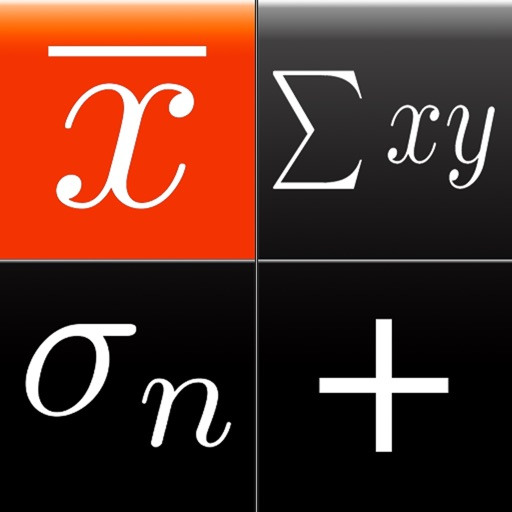Statistics Calculator++ is a scientific calculator with statistical and regression analysis capabilities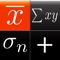Statistics Calculator++

by Dogahe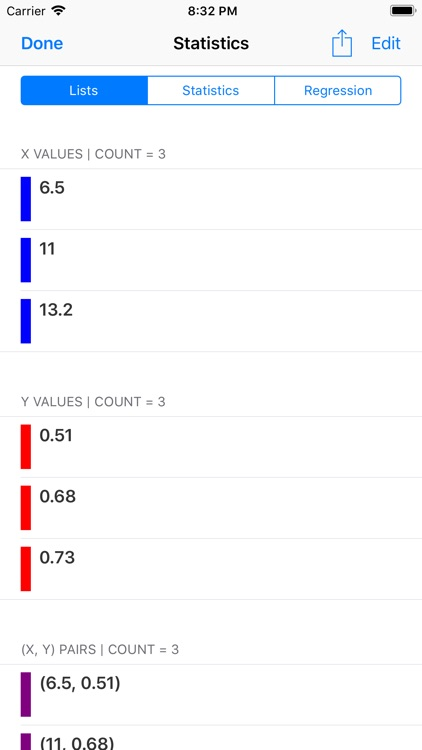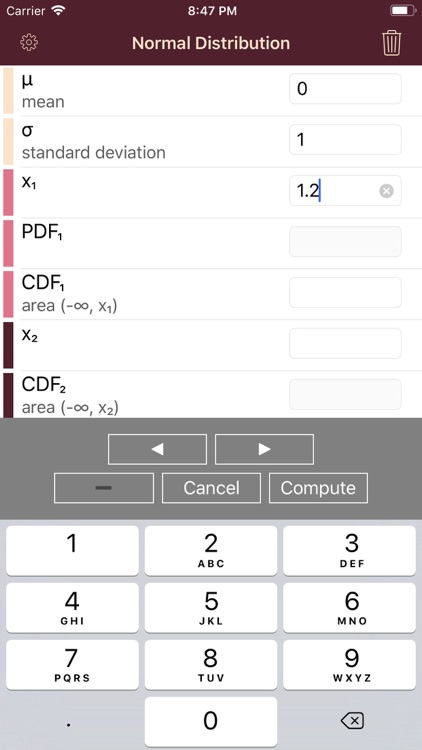What is it about?

Statistics Calculator++ is a scientific calculator with statistical and regression analysis capabilities. It can also graph the regression models through an extra purchase of an In-App Purchase.App Details

Version
1.38
Rating
(155)
Size
10Mb
Genre
Utilities Finance
Last updated
August 12, 2019
Release date
August 8, 2012

Statistics Calculator++ is \$1.99 but there are more add-ons

• \$0.99

Graphing Regression Models

• \$0.99

App Screenshots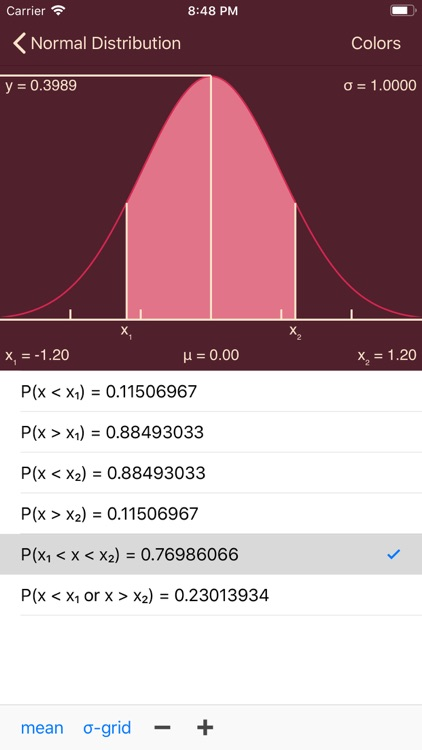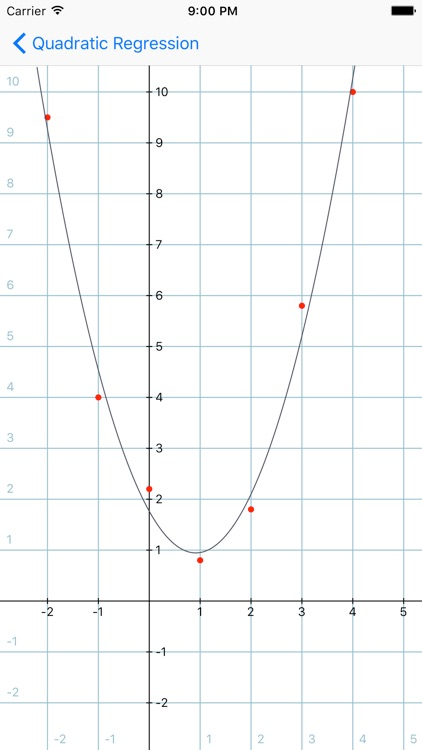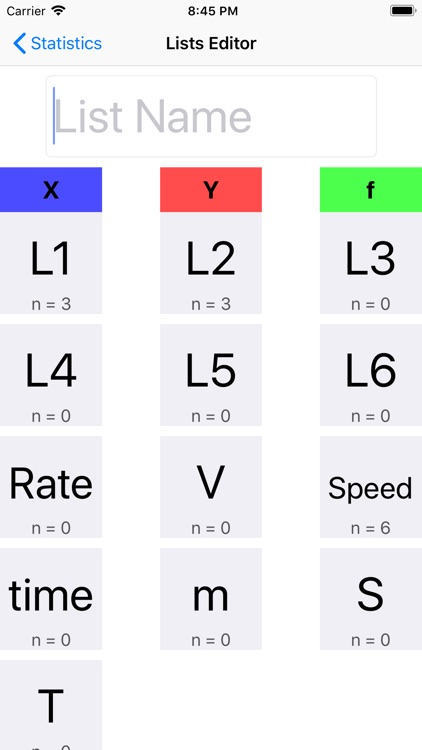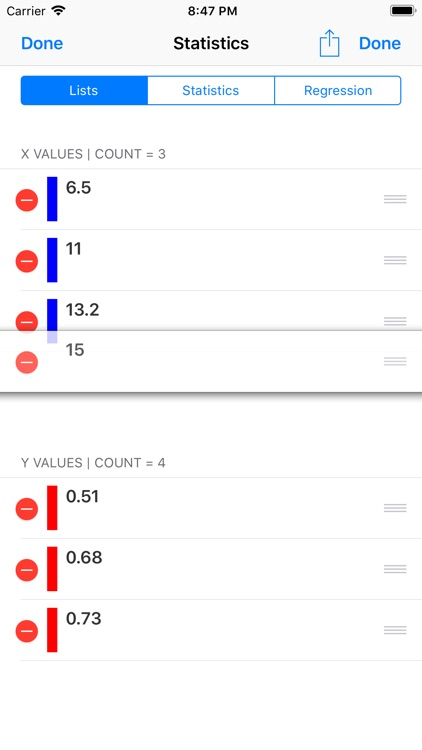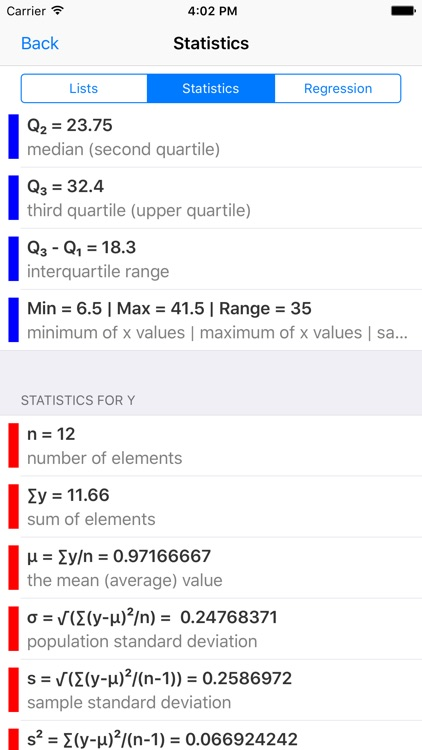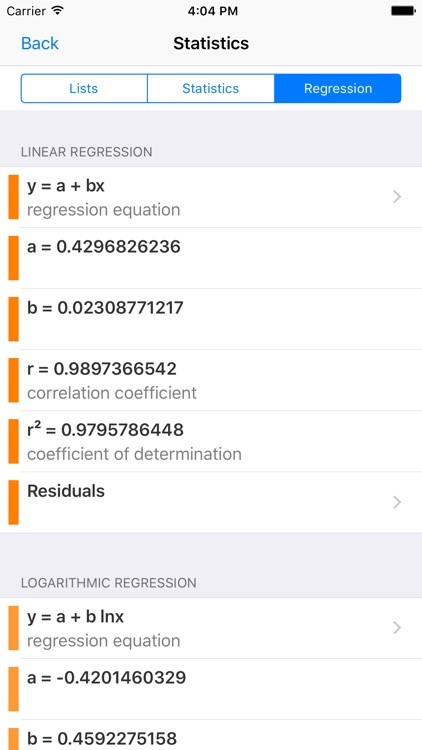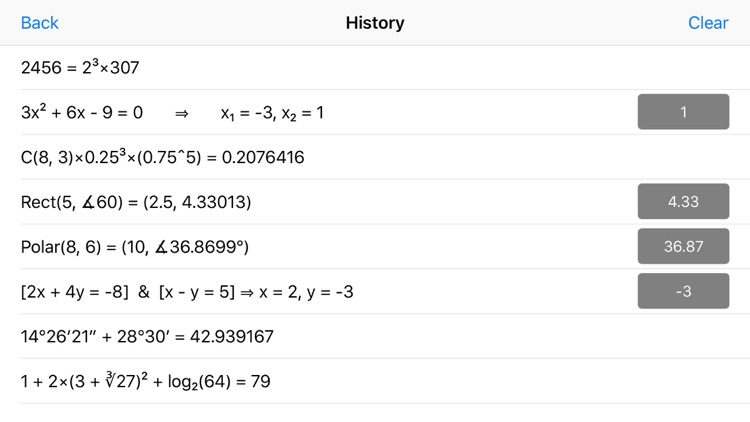App Store Description

Statistics Calculator++ is a scientific calculator with statistical and regression analysis capabilities. It can also graph the regression models through an extra purchase of an In-App Purchase.

In designing the statistics functions of this app we had one thing in mind: The user should not need to read a single line of manual to perform the statistical analysis.

Simply use Add X, Add Y, and Add f buttons to enter the elements of the lists and frequencies, if any. And you are done. All the statistics and regression models are only a few taps and swipes away. Tap the List button to see the lists entered. The elements of the lists are interpreted independently (as two separate lists) and jointly for regression analysis (if the size of the lists are equal).

The sum, mean, population standard deviation, sample standard deviation, sample variance, sum of squares, min, max, quartiles, and sum of multiplications of two lists are presented in a table. Each statistics measure can be chosen from the table and used for further calculations.

For regression analysis linear regression, logarithmic regression, exponential regression, power regression, and quadratic regression (In-App Purchase) are calculated. Statistics Calculator++ has an extremely simple and intuitive user interface to choose the regression model and calculate predictions for x and y values and view residuals and residual sum of squares.

You have the capability to add up to 12 lists and use any of them as list X, list Y, or list f. Access Lists Editor feature by tapping the icon on top right of the Statistics Lists view. Furthermore, all these lists persist in the memory. Therefore, if you close and the relaunch the app the lists are there for you.

Normal distribution functionality of TI-83 or TI-84 (normalpdf, normalcdf, invNorm, ShadeNorm), Student's t-distribution (tpdf, tcdf, invT), Chi-Squared distribution, F distribution, Binomial distribution (binompdf, binomcdf), Poisson distribution (poissonpdf, poissoncdf), and Geometric distribution (geometpdf, geometcdf) are all possible with this app with an easy to use, and beautiful user interface.

Other major features of Statistics Calculator++ are:

- The left pane scrolls to reveal more functionality without a need to switch modes. The digits and most common operations are always visible and the less common operations can be revealed by scrolling the left half of the screen.
- Shows the expression as you enter and keeps a history of your calculations (Swipe up on the screen to reveal the history)
- Capability of performing fraction manipulations and converting between different formats (proper, improper, and mixed fractions) and seamless integration of fraction, integer, and decimal manipulations.
- Capability of inputting degrees, minutes, and seconds for angles and converting between degrees-minutes-seconds format and decimal format.
- Capability of calculating permutation, combination, Least Common Multiple (LCM), Greatest Common Divisor (GCD), and prime factorization.
- Quadratic and system of two linear equations solver
- Rectangular to polar and polar to rectangular coordinate conversion (extremely useful for phasor calculations for electrical engineers)
- Throws a set of dice (choice of 1 die to 4 dice)
- Backspace to delete last digit entered.
- Sinusoidal functions can be calculated for angles in units of degrees, radians, or gradians.
- Showing the content of memory on MR button, as long as it fits.

Statistics Calculator++ is an ongoing project and we are working on more features and functions to be added to every update of the app.

You are going to love the simplicity of the user interface and yet have the powerful functionality with which Statistics Calculator++ is loaded.

Please do not hesitate to contact us for any questions, comments, to report any bugs, or to suggest additional functions you would like to see in the upcoming updates.

Disclaimer:
AppAdvice does not own this application and only provides images and links contained in the iTunes Search API, to help our users find the best apps to download. If you are the developer of this app and would like your information removed, please send a request to [email protected] and your information will be removed.